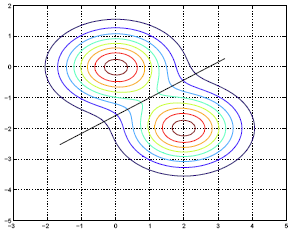# 9.2.4 - Binary ClassificationPrinter-friendly version

In binary classification in particular, for instance if we let (k =1, l =2), then we would define constant $a_0$, given below, where $\pi_1$ and $\pi_2$ are prior probabilities for the two classes and $\mu_1$ and $\mu_2$ are mean vectors.

• Binary classification (k = 1, l = 2):
• Define $a_0 =\text{log }\frac{\pi_1}{\pi_2}-\frac{1}{2}(\mu_1+\mu_2)^T\Sigma^{-1}(\mu_1-\mu_2)$
• Define $(a_1, a_2, ... , a_p)^T = \Sigma^{-1}(\mu_1-\mu_2)$
• Classify to class 1 if $a_0 +\sum_{j=1}^{p}a_jx_j >0$ ; to class 2 otherwise.
• An example

$\ast \pi_1=\pi_2=0.5$
$\ast \mu_1=(0,0)^T, \mu_2=(2,-2)^T$
$\ast \Sigma = \begin{pmatrix} 1.0&0.0 \\ 0.0 & 0.5625 \end{pmatrix}$
$\ast \text{Decision boundary: } 5.56-2.00x_1+3.56x_2=0.0$

Here is a contour plot of this result:We have two classes and we know the within class density. The marginal density is simply the weighted sum of the within class densities, where the weights are the prior probabilities. Because we have equal weights and because the covariance matrix two classes are identical, we get these symmetric lines in the contour plot. The black diagonal line is the decision boundary for the two classes. Basically, if you are given an x above the line, then  we would classify this x into the first-class. If it is below the line, we would classify it into the second class.

There is a missing piece here, right?

For all of the discussion above we assume that we have the prior probabilities for the classes and we also had the within class densities given to us. Of course, in practice you don't have this. In practice, what we have is only a set of training data.

The question is how do we find the $\pi_k$'s and the $f_k(x)$?